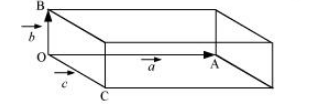# Show that $\mathbf{a} .(\mathbf{b} \times \mathbf{c})$ is equal in magnitude to the volume

Question.
Show that $\mathbf{a} .(\mathbf{b} \times \mathbf{c})$ is equal in magnitude to the volume of the parallelepiped formed on the three vectors, $\mathbf{a}, \mathbf{b}$ and $\mathbf{c}$.

solution:

A parallelepiped with origin O and sides a, b, and c is shown in the following figure.Volume of the given parallelepiped = abc

$\overrightarrow{\mathrm{OC}}=\vec{a}$

$\overrightarrow{\mathrm{OB}}=\vec{b}$

$\overrightarrow{\mathrm{OC}}=\vec{c}$

Let $\hat{\mathbf{n}}$ be a unit vector perpendicular to both $b$ and $c$. Hence, $\hat{\mathbf{n}}$ and a have the same direction.

$\therefore \vec{b} \times \vec{c}=b c \sin \theta \hat{\mathbf{n}}$

$=b c \sin 90^{\circ} \hat{\mathbf{n}}$

$=b c \hat{n}$

$\vec{a} \cdot(\vec{b} \times \vec{c})$

$=a \cdot(b c \hat{\mathbf{n}})$

$=a b c \cos \theta \hat{\mathbf{n}}$

$=a b c \cos 0^{\circ}$

$=a b c$

= Volume of the parallelepiped